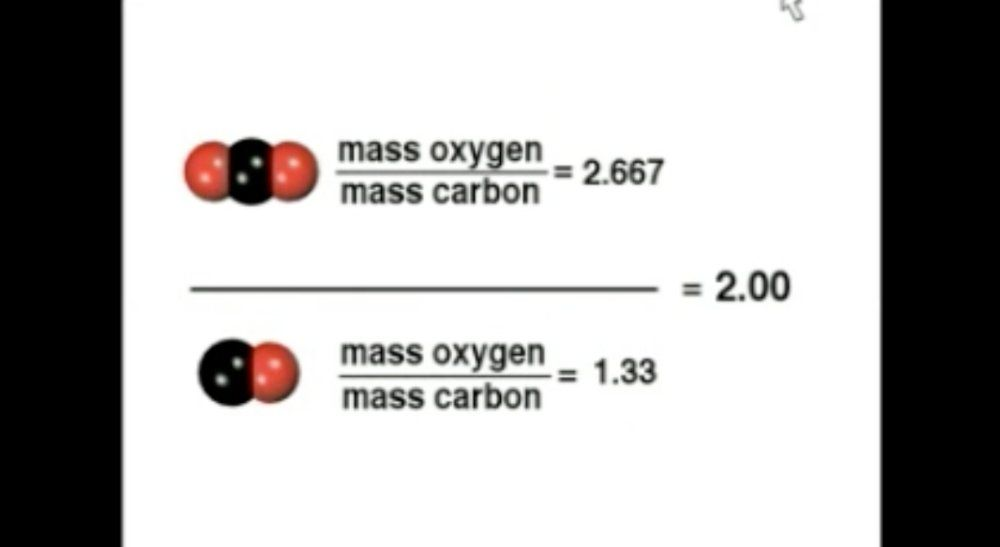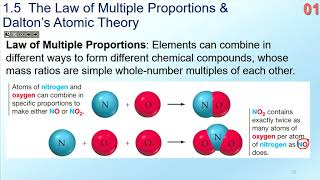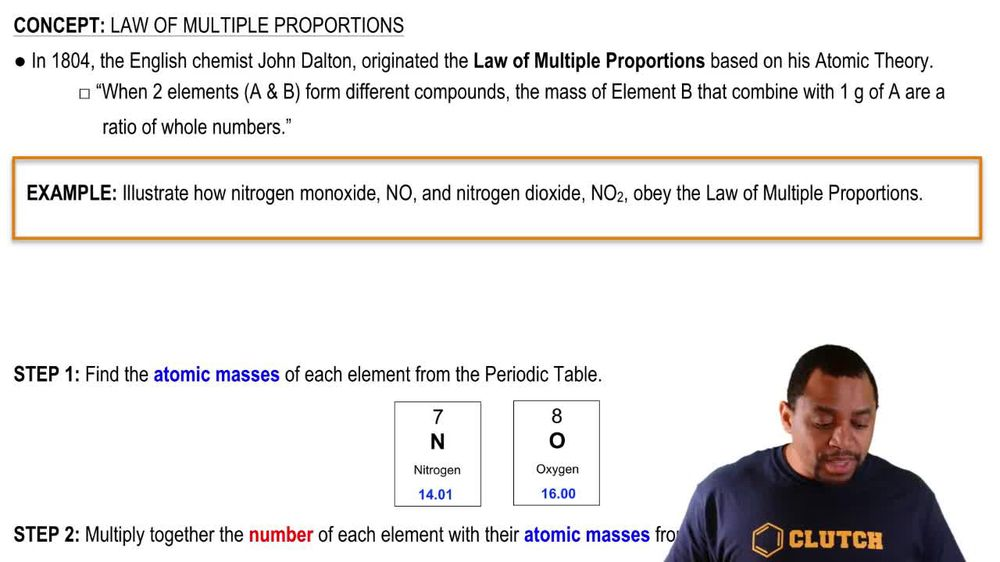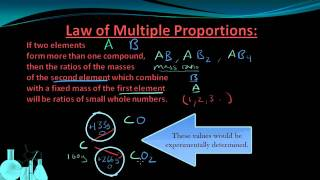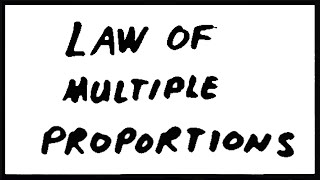Start typing, then use the up and down arrows to select an option from the list.
1. 2. Atoms & Elements2. Law of Multiple Proportions
Problem

# What is the law of multiple proportions, and how does Dalton’s atomic theory account for it?

Relevant Solution2m
Play a video:
Hey everyone in this example, we need to choose among the statements below as to which one demonstrates the law of multiple proportions in the truest way. And we need to explain our answer. So let's analyze the first choice, which says that hydrogen peroxide. So that's going to be a church to 02 has a mass ratio of 16 to 1 for oxygen to hydrogen. and so this ratio here is 16-1. They likely get from the massive hydrogen where it's When we recall to the periodic table, we get a massive 2g for two atoms of hydrant and then for the massive oxygen for two atoms of oxygen, we have a mass for each atom equal to a total of 32 g. And so they simplify this so that it's up, it's up to being a ratio of 1 to 16 when we divide two x 2 and 32 x two. So we get 1-16. And so they actually just flipped the ratio. So they wrote it for oxygen to hydrogen so that we have 16-1. And so for the second compound, they say water, H20 has a mass ratio Of oxygen to hydrogen being a value of 8 : one. And so they likely got that by looking at the mass of the two hydrogen atoms in the compound. So again, that's equal to two g. When we recall from the periodic table. And then for the single atom of oxygen in this compound, we have a mass of 16 g. And so they simplify this yet again by dividing two by two and then two by So that they end up with 1-8. And so they actually flip the ratio so that it's for oxygen to hydrogen as 8-1. And so this definitely does match up with our definition for the law of multiple proportions, which says that different compounds will have defined ratios and we do have these whole number defined ratios between each of these compounds with similar atoms. So we can actually go ahead and rule out all the other answer choices because we already have our correct answer choice as choice A So this will complete this example as our final answer, which is the only statement below that demonstrates the law of multiple proportions. So I hope that everything we reviewed was clear. If you have any questions, please leave them down below and I will see everyone in the next practice video.